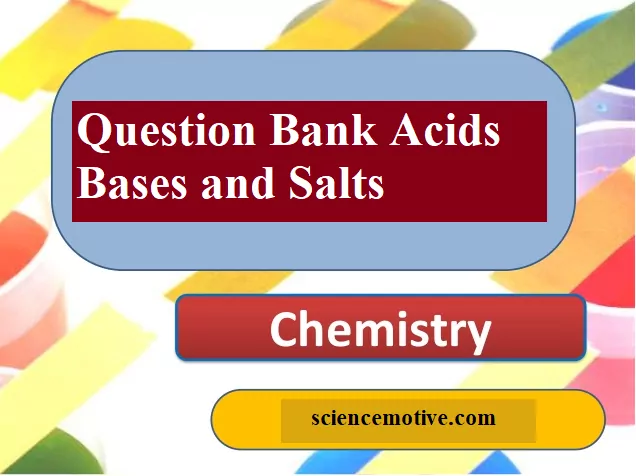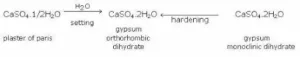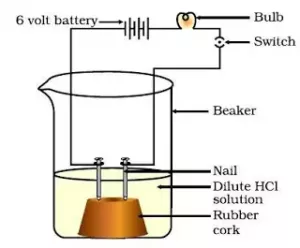# Question Bank Acids Bases and SaltsQuestion Bank Acids Bases and Salts

Que 1. Define the terms: Acid, alkali and salt.
Ans 1. An acid is a compound, which releases hydronium ions (H3O+) as the only positive ions in solution.
An alkali is a compound, which releases hydroxyl ions (OH) as the only negative ions in solution.
Salt is one of the products of neutralization between an acid and a base; water being the only other product.
OR
A salt gives positive ions other than H+ ion and negative ions other than OH ion in solution.

Que 2. Identify the number of replaceable hydrogen ions (H+) in the following acids: HCl, CH3COOH, H2SO4, H3PO4.
Ans 2. HCl = 1     CH3COOH = 1         H2SO4 = 2       H3PO4 = 3.

Que 3. What is a neutralization reaction?
Ans 3. Neutralization is essentially a chemical reaction between H3O+ ions of an acid with OH ions of the base, to give undissociated molecules of water.

Que 4. What are strong and weak acids? Give one example of each?
Ans 4. A strong acid is one, which is almost completely dissociated in the solution.
Examples: Dilute nitric acid, dilute sulphuric acid and dilute hydrochloric acid. A weak acid is one, which is only partially ionized in solution (degree of dissociation is >30%). Examples: Acetic acid, carbonic acid, and sulphurous acid.

Que 5. What is the common name of the compound CaOCl2?
Ans 5. The common name of the compound CaOCl2 is bleaching powder.

Question Bank Acids Bases and Salts

Que 6. Name the substance which on treatment with chlorine yields bleaching powder?
Ans 6. Calcium hydroxide [Ca(OH)2], on treatment with chlorine, yields bleaching powder.

Que 7. Name the sodium compound which is used for softening hard water.
Ans 7. Washing soda (Na2CO3.10H2O) is used for softening hard water.

Que 8. What will happen if a solution of sodium hydrogen carbonate is heated? Give the equation of the reaction involved.
Ans 8. When a solution of sodium hydrogen carbonate is heated, sodium carbonate and water are formed with the evolution of carbon dioxide gas.
NaHCO3 → Na2CO3 + H2O + CO2 ↑

Que 9. Write an equation to show the reaction between Plaster of Paris and water.
Ans 9. The chemical equation for the reaction of Plaster of Paris and water can be represented asQue 10. Why acetic acid is called a weak acid though there are 4 ‘H’ atoms in the molecule?
Ans 10. Acetic acid is called a monobasic acid because only one of the 4 ‘H’ atoms of the acid is released as an H+ ion in solution.

Question Bank Acids Bases and Salts

Que 11. How does a strong acid differ from a concentrated acid?
Ans 11. The strength of an acid depends upon its dissociation power whereas concentration depends on the water content in the acid.

Que 12. Why is Plaster of Paris written as CaSO4.½H2O? How is it possible to have half a water molecule attached to CuSO4?
Ans 12. The actual formula of Plaster of Paris is 2CaSO4.H2O which means that one molecule of H2O is associated with two molecules of CaSO4. The formula for simplicity is written as CaSO4. ½H2O.

Que 13. Name a salt of a strong acid HNO3 and a weak base like NH4OH. Represent the reaction that takes place.
Ans 13. The salt that results due to the above reaction is Ammonium nitrate (NH4NO3). The reaction is represented as:
HNO3 + NH4OH →  NH4NO3 + H2O

Que 14. i) Name a strong base and a weak base.
ii) Name a hydrated salt.
Ans 14. i) A strong base is sodium hydroxide (NaOH) and a weak base is ammonium hydroxide (NH4OH).
ii) A hydrated salt is copper sulphate crystals (CuSO4.5H2O).

Que15. A solution turns red litmus blue, its pH is likely to be
(a) 1         (b) 4          (c) 5         (d) 10
Ans 15. (d) Bases turn red litmus blue and acids turn blue litmus red. The basic solution has a pH value more than 7. Since the solution turns red litmus blue, its pH is likely to be 10.

Question Bank Acids Bases and Salts

Que 16. A solution reacts with crushed egg-shells to give a gas that turns lime-water milky. The solution contains
(a) NaCl             (b) HCl             (c) LiCl             (d) KCl
Ans 16. (b) The solution contains HCl.

Que 17. 10 mL of a solution of NaOH is found to be completely neutralised by 8 mL of a given solution of HCl. If we take 20 ml of the same solution of NaOH, the amount of HCl solution (the same solution as before) required to neutralise it will be
(a) 4 mL         (b) 8mL         (c) 12 mL     (d) 16 mL
Ans 17. (d) 16 mL of HCl solution will be required.

Que 18. Which one of the following types of medicines are used for treating indigestion? Antibiotic (b) Analgesic (c) Antacid (d) Antiseptic
Ans 18. (c) An antacid is used for treating indigestion.

Que 19. Name the following:
a) Two non-hydrated crystalline salts
b) Two neutral salts
c) Two basic salts
d) Two acid salts
Ans 19. a) Two non-hydrated crystalline salts are: sodium chloride (NaCl) and potassium nitrate (KNO3)
b) Two neutral salts are: sodium chloride (NaCl) and sodium sulphate(Na2SO4)
c) Two basic salts are: basic copper carbonate (CuCO3.Cu(OH)2) and basic lead carbonate(PbCO3.Pb(OH)2)
d) Two acid salts are sodium bicarbonate (NaHCO3) and sodium phosphate (NaH2PO4).

Que 20. Name the salts of sulphuric acid.
Ans 20. The salts of sulphuric acid are bisulphate and sulphate. Examples: NaHSO4, KHSO4 and Na2SO4.

Question Bank Acids Bases and Salts

Que 21. Compounds such as alcohols and glucose also contain hydrogen but are not categorized as acids. Describe an activity to prove it.
Ans 21. Two nails are fitted on a cork and are kept in a 100 mL beaker. The nails are then connected to the two terminals of a 6-volt battery through a bulb and a switch. Some dilute HCl is poured into the beaker and the current is switched on. The same experiment is then performed with a glucose solution and an alcohol solution.Result: HCl dissociates into H+ and Cl ions. These ions conduct electricity in the solution resulting in the glowing of the bulb. On the other hand, the glucose solution does not dissociate into ions. Therefore, it does not conduct electricity.
Conclusion: From this activity, it can be concluded that all acids contain hydrogen but not all compounds containing hydrogen are acids.
That is why, though alcohols and glucose contain hydrogen, they are not categorised as acids.

Que 22. Why does distilled water not conduct electricity, whereas rainwater does?
Ans 22. Distilled water is a pure form of water and is devoid of any ionic species. Therefore, it does not conduct electricity. Rainwater, being an impure form of water, contains many ionic species such as acids and therefore it conducts electricity.

Que 23. Why do acids not show acidic behaviour in the absence of water?
Ans 23. Acids do not show acidic behaviour in the absence of water because the dissociation of hydrogen ions from an acid occurs in the presence of water only. It is the hydrogen ions that are responsible for the acidic behaviour.

Que 24. Equal lengths of magnesium ribbons are taken in test tubes A and B. Hydrochloric acid (HCl) is added to test tube A, while acetic acid (CH3COOH) is added to test tube B. In which test tube will the fizzing occur more vigorously and why?
Ans 24. The fizzing will occur strongly in test tube A, in which hydrochloric acid (HCl) is added. This is because HCl is a stronger acid than CH3COOH and therefore produces hydrogen gas at a faster speed due to which fizzing occurs.

Que 25. Fresh milk has a pH of 6. How do you think the pH will change as it turns into curd? Explain your answer.
Ans 25. The pH of milk is 6. As it changes to curd, the pH will reduce because curd is acidic in nature. The acids present in it decrease the pH.

Question Bank Acids Bases and Salts